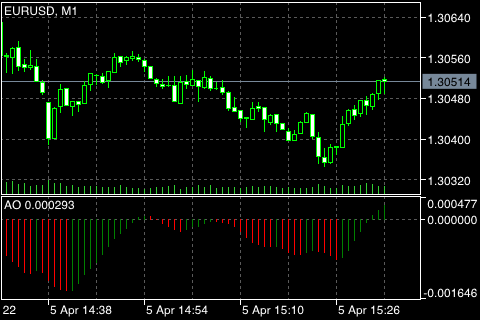# Awesome Oscillator

Bill Williams's Awesome Oscillator Technical Indicator (AO) is a 34-period simple moving average, plotted through the middle points of the bars (H+L)/2, which is subtracted from the 5-period simple moving average, built across the central points of the bars (H+L)/2. It shows us quite clearly whats happening to the market driving force at the present moment.

"Saucer" is the only signal to buy that comes when the histogram is above the zero line. It is necessary to remember the following:

• the saucer signal is generated when the histogram reversed its direction from the downward to upward. The second column is lower than the first one and is colored red. The third column is higher than the second and is colored green;
• for the saucer signal to be generated, the histogram should have at least three bars.

Keep in mind, that all Awesome Oscillator bars should be above the zero line for the saucer signal to be used.

"Zero Line Cross" is a Buy signal formed when the histogram moves from negative to positive values. Wherein:

• for this signal to be generated, only two bars are necessary;
• the first bar is to be below the zero line, the second one is to cross it (transition from a negative value to a positive one);
• simultaneous generation of buy and sell signals is impossible.

"Twin peaks" – is the only Buy signal that can be generated when the histogram values appear below zero. It is necessary to remember the following:

• the signal is generated, when you have a peak pointing down (the lowest low) which is below the zero line and is followed by another down-pointing peak which is somewhat higher (a negative figure with a lesser absolute value, which is therefore closer to the zero line), than the previous down-looking peak;
• the histogram is to be below the zero line between the two peaks. If the histogram crosses the zero line in the section between the pikes, the signal to buy does not function. However, a different signal to buy will be generated – Zero Line Cross;
• each new peak of the histogram is to be higher (a negative number of a lesser absolute value that is closer to the zero line) than the previous peak;
• if an additional higher peak is formed (that is closer to the zero line) and the histogram has not crossed the zero line, an additional buy signal will be generated.

## Sell Signals

Awesome Oscillator sell signals are identical to buy. The Saucer signal is reversed as is below zero. Zero Line Cross is on the decrease – the first bar of it is above zero, the second one is below it. The Twin Peaks is above the zero line and is reversed too.## Calculation

AO is a 34-period simple moving average, plotted through the median values of the bars (H+L)/2, and subtracted from the 5-period simple moving average, built across the median points of the bars (H+L)/2.

MEDIAN PRICE = (HIGH + LOW) / 2

AO = SMA (MEDIAN PRICE, 5) - SMA (MEDIAN PRICE, 34)

Where:

MEDIAN PRICE – the median price;
HIGH – the high price of the bar;
LOW – the low price of the bar;
SMA – Simple Moving Average.